• 密度图如何绘制
2021-11-12 22:14:44

教程文档下载.pdf

交 流 分 享 扣 扣 群 ： 925920448 \color{red}{交流分享扣扣群：925920448}r语言 密度曲线
更多相关内容
• CNN-人群密度检测-密度图制作-数据集-标注工具等，这里的内容主要是本人在学习过程中在网络上收集所得。也有部分自己的改写。费了半天劲儿整理。绝对物超所值。
• 本文绘制这种图：每个SNP在染色体上的分布图，也称为SNP密度图，不同的颜色表示1Mb内包含的SNP个数。

本文绘制这种图：

每个SNP在染色体上的分布图，也称为SNP密度图，不同的颜色表示1Mb内包含的SNP个数。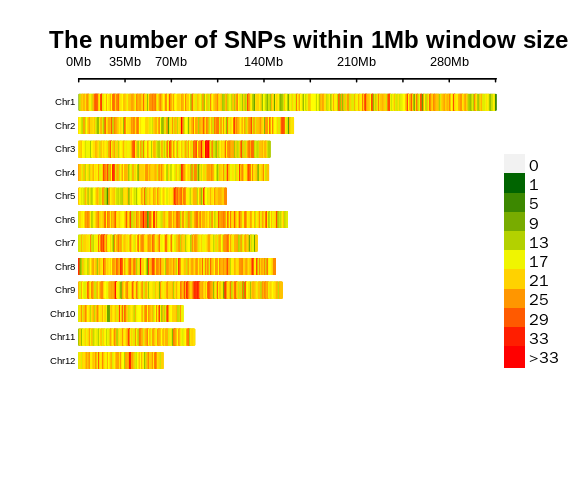## 用到的R包CMplot

安装方法：

install.packages("CMplot")


## 数据格式

1 1_320344 0 320344
1 1_342499 0 342499
1 1_509942 0 509942
1 1_538165 0 538165
1 1_565638 0 565638
1 1_612572 0 612572
1 1_722644 0 722644
1 1_791066 0 791066
1 1_813662 0 813662
1 1_865366 0 865366



也可以只包括三列数据：

• 染色体
• SNP名称
• 物理位置

## 代码

library(data.table)
library(CMplot)

mm = map1 %>% dplyr::select(SNP = 2,Chromosome=1,Position = 4)

CMplot(mm,plot.type="d",bin.size=1e6,col=c("darkgreen", "yellow", "red"),
file="tiff",memo="",dpi=300,file.output=TRUE, verbose=TRUE)

CMplot(mm,plot.type="d",bin.size=1e6,col=c("darkgreen", "yellow", "red"),
file="tiff",memo="",dpi=300,file.output=FALSE, verbose=TRUE)


## 结果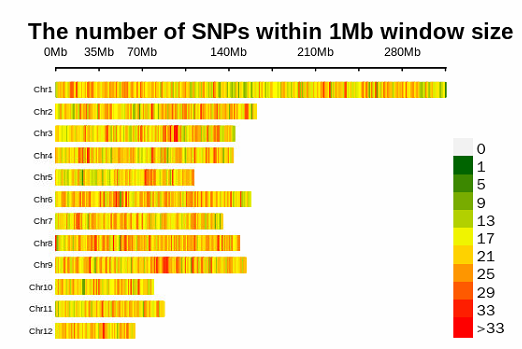很简单有没有！！！

展开全文python
• 双重差分模型理论讲解学习笔记（还讲了三重差分的实现原理），以及配套的DID代码+数据，PSM-DID,动态效应检验，核密度图绘制，跟着我整理的学习笔记学习即可快速掌握DID的原理以及操作的核心要义，里面的理论介绍...
• 今天小编就为大家分享一篇python绘制直方图和密度图的实例，具有很好的参考价值，希望对大家有所帮助。一起跟随小编过来看看吧
• DDMaker执行局部密度分析，并从RGB颜色，灰度或二进制图像开始，生成2D图像中成像的细胞结构的空间分布的伪彩色。 DDMaker局部密度分析允许选择性地对信号进行降噪，可视化和量化其分布，并基于局部密度对图像进行...
• 直方图和核密度图 1 pandas 方法 import pandas as pd import matplotlib.pyplot as plt # 不能漏掉.pyplot data=pd.read_csv(r’C:\01Pylearn\data_for_analysis\table4-3.csv’, sep=’,’, engine=‘python’, ...

## 直方图和核密度图

1 pandas 方法
import pandas as pd
import matplotlib.pyplot as plt # 不能漏掉.pyplot
data=pd.read_csv(r’C:\01Pylearn\data_for_analysis\table4-3.csv’, sep=’,’, engine=‘python’, encoding=‘utf-8’)
#分割符错误会导致问题
data
data.Age.plot(kind=‘hist’, bins=20, color=‘blue’, edgecolor=‘black’, density= True, label= “直方图”) # normed不再使用，更新为density
data.Age.plot(kind=‘kde’, color = ‘red’, label=‘核密度图’)
plt.xlabel(‘年龄’)
plt.ylabel(‘核密度’)
plt.title(‘年龄分布图’)
plt.legend()
#中文符号显示问题
plt.rcParams[‘font.sans-serif’]=[‘SimHei’]
plt.show()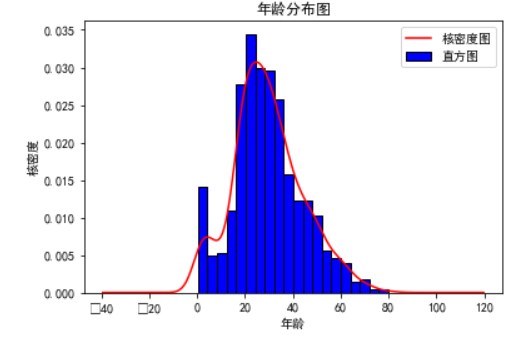import numpy as np
import pandas as pd
import matplotlib.pyplot as plt
import seaborn as sns

data =pd.read_csv(r’C:\01Pylearn\data_for_analysis\table4-3.csv’, sep=’,’, engine=‘python’, encoding=‘utf-8’)
data
Age_Male= data.Age[data.Sex==‘male’]
Age_Male_dropna=Age_Male.dropna() #需要清理没有数据的

Age_Female= data.Age[data.Sex==‘female’]
Age_Female_dropna=Age_Female.dropna()

sns.distplot(Age_Male_dropna, bins=20, kde= False, hist_kws = {‘color’:‘blue’}, label=‘男性’)
sns.distplot(Age_Female_dropna, bins=20, kde= False, hist_kws = {‘color’:‘red’}, label=‘女性’)

plt.title(‘年龄分布图’)
plt.legend()
#中文符号显示问题
plt.rcParams[‘font.sans-serif’]=[‘SimHei’]
plt.show()

sns.distplot(Age_Male_dropna,hist= False, kde_kws = {‘color’:‘blue’, ‘linestyle’:’-’},norm_hist= True,label=‘男性’)
sns.distplot(Age_Female_dropna,hist= False, kde_kws = {‘color’:‘blue’, ‘linestyle’:’–’},norm_hist= True,label=‘女性’)
plt.title(‘年龄核密度图’)
plt.legend()
#中文符号显示问题
plt.rcParams[‘font.sans-serif’]=[‘SimHei’]
plt.show()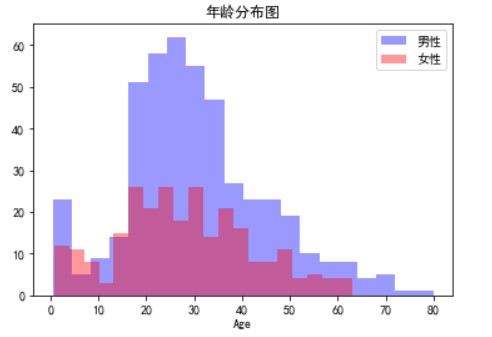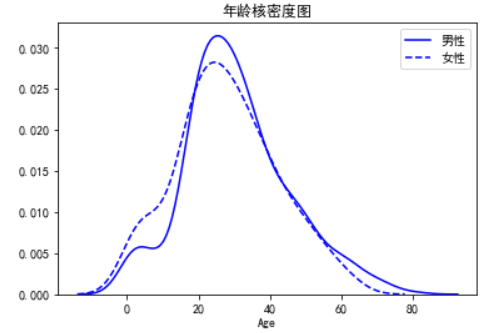展开全文• 本文件包含详细路网分布与密度专题的技术分布与操作录屏，非常适合想使用ArcGIS制作专题的初学者！
• 目录   1、scipy库中各分布对应的方法   2、stats中各分布的常用方法及其功能   3、正态分布的概率密度函数及其图象 ...    3）python绘制t分布和正态分布的概率密度函数对比   6、F分布的概率密度函数
• 我想做一个三维的散点云图，云图颜色能够表示数据的疏密程度，不知道各位有没有好的方法可以实现，目前我只会2维的。matlab
• 当我们想要弄清楚变量的统计特性时，往往想知道它是服从什么分布的，这时候就需要绘制概率分布直方图 ...在seaborn中的distplot函数可以完成概率分布直方图和密度图绘制 seaborn.distplot(a, bins=None, his.

当我们想要弄清楚变量的统计特性时，往往想知道它是服从什么分布的，这时候就需要绘制概率分布直方图

在python中我们可以使用seaborn库来进行绘制：

Seaborn是一个基于matplotlib的Python数据可视化库。它为绘制有吸引力和信息丰富的统计图形提供了高级界面。

首先需要导入seaborn库：

import seaborn as sns


在seaborn中的distplot函数可以完成概率分布直方图和密度图的绘制

seaborn.distplot(a, bins=None, hist=True, kde=True, rug=False,
fit=None, hist_kws=None, kde_kws=None, rug_kws=None, fit_kws=None,
color=None, vertical=False, norm_hist=False, axlabel=None,
label=None, ax=None)


下面直接给出我绘制时用到的代码：

import seaborn as sns
import matplotlib as mpl
import matplotlib.pyplot as plt
from scipy.stats import norm
from scipy.stats import laplace

txt=[]
data=[]
path = r"E:\rtklib\rtklib-test1\stav.txt"

#mpl.rc("figure", figsize=(9, 5))
with open(path, "r") as f:

for i in range(len(txt)):
data.append(float(txt[i].strip().split(',')))

sns.set_palette("hls")
#sns.set_style("whitegrid")
plt.figure(dpi=120)
sns.set(style='dark')
sns.set_style("dark", {"axes.facecolor": "#e9f3ea"})
g = sns.distplot(data,
hist=True,
kde=True,  # 开启核密度曲线kernel density estimate (KDE)
kde_kws={'linestyle': '--', 'linewidth': '1', 'color': '#c72e29',
# 设置外框线属性
},
fit=norm,
color='#098154',
axlabel='Standardized Residual',  # 设置x轴标题

)

plt.show()


其中，distplot的参数中，kde表示是否绘制核密度曲线；fit是选择拟合的分布，来分析数据究竟是符合什么分布，seaborn中提供了很多分布，可以在这里找到，使用时如上我的代码中导入的那样就可以：连续分布类型
最后的效果图如下：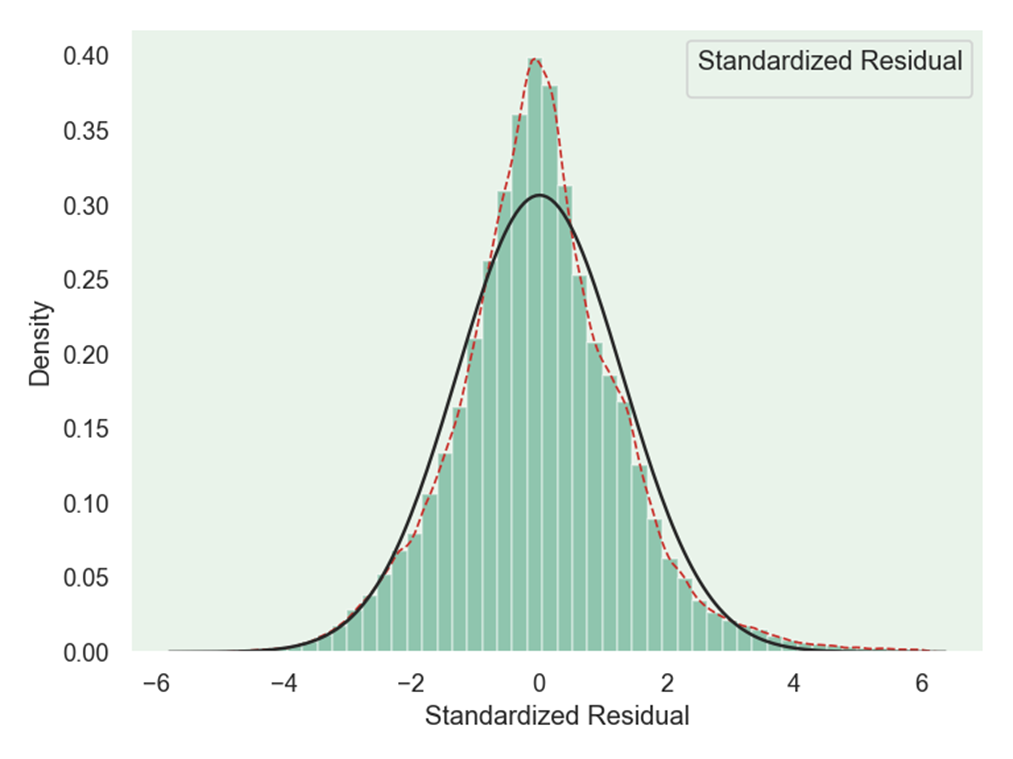绘制过程中没有加入太多修饰，如果想要好看一点，可以修改一些参数：
其中一个有设置背景 的风格，主要有以下几种：

sns.set_style("whitegrid")
sns.set_style("dark")
sns.set_style("darkgrid")
sns.set_style("white")
sns.set_style("ticks")

展开全文python
• figure plot(r,p1,r,p2) legend('{\mu=0.5，\sigma=0.5}','{\mu=0.5，\sigma=1}') xlabel('接受信号包络','fontsize',12) ylabel('概率密度函数','fontsize',12) grid on set(gcf,'color','w'); 4、参考论文 略
• ## 绘制自己的密度图

千次阅读 热门讨论 2019-04-17 19:45:12
主要用Matlab就搞定了 1、人头标定 ...修改76、77行，新建density_map文件夹保存生成的密度图，不过自动保存的密度图是.fig文件，不如自己手动保存为.jpg好用     成功结束。。
• 由于过度绘制，可能很难从散点中读取数据密度。 因此，我将每个像素的数据密度计算为与每个点的距离平方和的倒数，并添加一个模糊因子以防止像素内的实际点变为无穷大。 结果允许对数据密度进行视觉修复。matlab
• ## Matlab绘制散点密度图

万次阅读 多人点赞 2020-11-01 16:54:24
使用Matlab绘制散点密度图，代码简单（附代码）。matlab
• ## 核密度图的绘制

千次阅读 2021-04-21 10:45:50
原标题：核密度图绘制我们先看一下别人家文章中的核密度图。 那么，怎么做出这样的图呢？这种核密度图到底想表达或表现什么呢？核密度图绘制所谓“眼过千遍不如手过一遍”，数据我们这里用Wikipedia文中的这6个...matlab核密度
• 上一篇文章写到如何利用ArcGIS...这篇文章来点不一样的，也是我感觉挺有意思的，就是利用ArcGIS绘制一种与众不同的人口分布密度图；在学习本文内容之前呢，小伙伴们首先得会利用ArcGIS创建渔网，需要上一篇基础上 ...
• 密度图 给定一个数据集，需要观察这些样本的分布情况，往往我们会采用直方图的方法来进行直观的展现。该方法简单，容易计算，但是直方图存在着如下的两大问题： 绘制直方图时，需要确定分组问题，如果分组不同，...
• 研究生期间写的绘制两幅图像的散点密度图，从蓝色到红色密度逐渐增大，论文绘图简单好用，只需要输入两幅图像即可
• 绘制 SNP 密度图 一般使用 CMplot 绘制SNP密度图： 包链接：https://github.com/YinLiLin/R-CMplot 要绘制 SNP 密度图，仅仅需要三列即可： a. 第一列是 SNP 名称 b. 第二列是染色体 c. 第三列是 SNP 的位置 d. 第四...
• ## python绘制密度图

千次阅读 2021-05-06 10:38:22
import pandas as pd df = pd.read_csv(r'C:\Users\zhoutao\Desktop\data.csv') df = df[['label']] #2000-8000为范围 df.dropna().plot(kind='kde', xlim=(2000,8000))
• ## ggplot2绘制密度图

千次阅读 2022-05-13 14:51:55
ggplot2绘制密度图 密度图可以展示数值型变量的数据分布，在ggplot2中可以使用geom_dendity（）功能来实现绘图 1.基础密度图 密度图绘制只需要输入一个数值型的向量，即可以绘制密度图 require(ggplot2)#加载绘图R...r语言
• 散点密度图的效果如下： 画这样的图可以用scatplot函数即可，这个函数不是MATLAB自带的函数，是MATLAB论坛上提供的，链接在文尾。 函数用法 out = scatplot(x,y,method,radius,N,n,po,ms) out = scatplot(x,y,...MATLAB MATLAB绘图
• matlab制作散斑图的程序，可以在窗口输入散斑大小和密度...